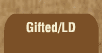Mathematics and Dyslexia

Tips for Learning the Times Tables

Tools for the Times Tables & Workbook

Home > Math > times tables practice > Higher nines times tables (scrambled)

## The Nines Times Tables - second set

The digits of the numbers that you can divide by nine always add up to nine. For 18, 1 + 8 is 9; for 27, 2 + 7 is 9, etc. etc.

Practice this page until they are fast and easy. This page should be as fast and easy as the 5s or the 1s. If you're having to stop and think a lot here, it won't get easier when these are mixed up with other numbers!

1. 9 x 9=
2. 9 x 6=
3. 9 x 10=
4. 9 x 7=
5. 9 x 8=
6. 8 x 9=
7. 6 x 9=
8. 9 x 6=
9. 9 x 9=
10. 7 x 9=
11. 6 x 9=
12. 9 x 8=
13. 8 x 9=
14. 9 x 6=
15. 10 x 9=